# Synthetic division of polynomial

Synthetic division of polynomial

Richard sells apples.

The previous day, his profits were $$$x$$, and today, his profits are$$$((x\times x)- 2)$$.

If the number of apples he sold was $$x+2$$, what was the profit made per apple?

We obtain the solution by modelling the equation as $$(x^2+x-2)\div (x+2)$$.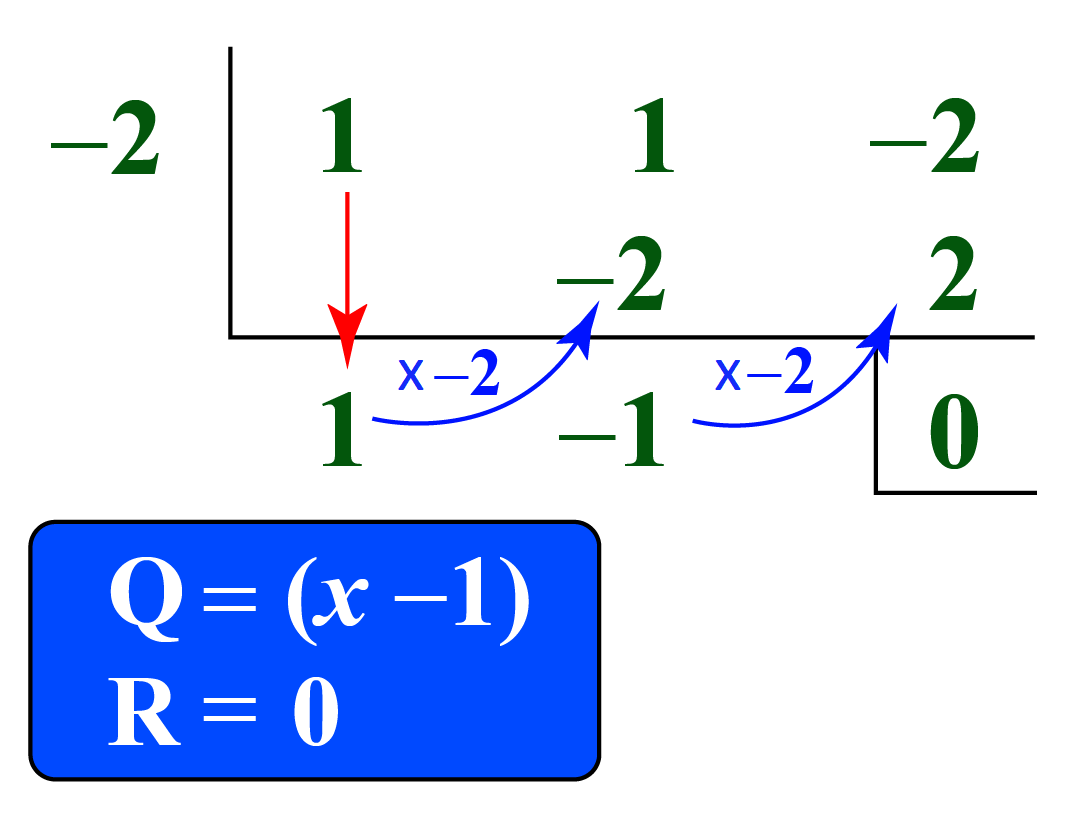The profit per apple is given by \$$$(x-1)$$

Synthetic division is the shorter method of the traditional long-division of a polynomial.

In this mini-lesson, we will learn about the synthetic division of polynomials. We will learn the synthetic division steps through many examples. We will also learn to use the synthetic division of polynomials calculator.

## Lesson Plan

 1 What Do You Mean by Synthetic Division of Polynomials? 2 Important Notes on Synthetic Division of Polynomials 3 Solved Examples on Synthetic Division of Polynomials 4 Interactive Questions on Synthetic Division of Polynomials

## What Do You Mean by Synthetic Division of Polynomials?

### Synthetic Division Method

When we divide a polynomial $$p(x)$$ by a linear factor $$x - a$$ (which is a polynomial of degree 1), $$Q(x)$$ is the quotient polynomial and $$R$$ (the constant term) is the remainder.

\begin{align}\dfrac{p(x)}{q(x)} &= \dfrac{p(x)}{(x - a)}\\\\&=Quotient + \dfrac{Remainder}{(x-a)}\end{align}

 $$\dfrac{p(x)}{(x-a)} = Q(x) + \dfrac{R}{x-a}$$

The coefficients of $$p(x)$$ are taken and divided by the zero of the linear factor.

We use synthetic division in the context of the evaluation of the polynomials by the remainder theorem, wherein we evaluate the value of $$p(x)$$ at $$a$$ while dividing $$\dfrac{p(x)}{(x-a)}$$.

That is, to find if $$a$$ is the factor of the polynomial $$p(x)$$, use the synthetic division to find the remainder quickly.

### Synthetic Division of Polynomials vs Long Division of polynomials

Let us see how long division differs from synthetic division of polynomials by comparing both the methods.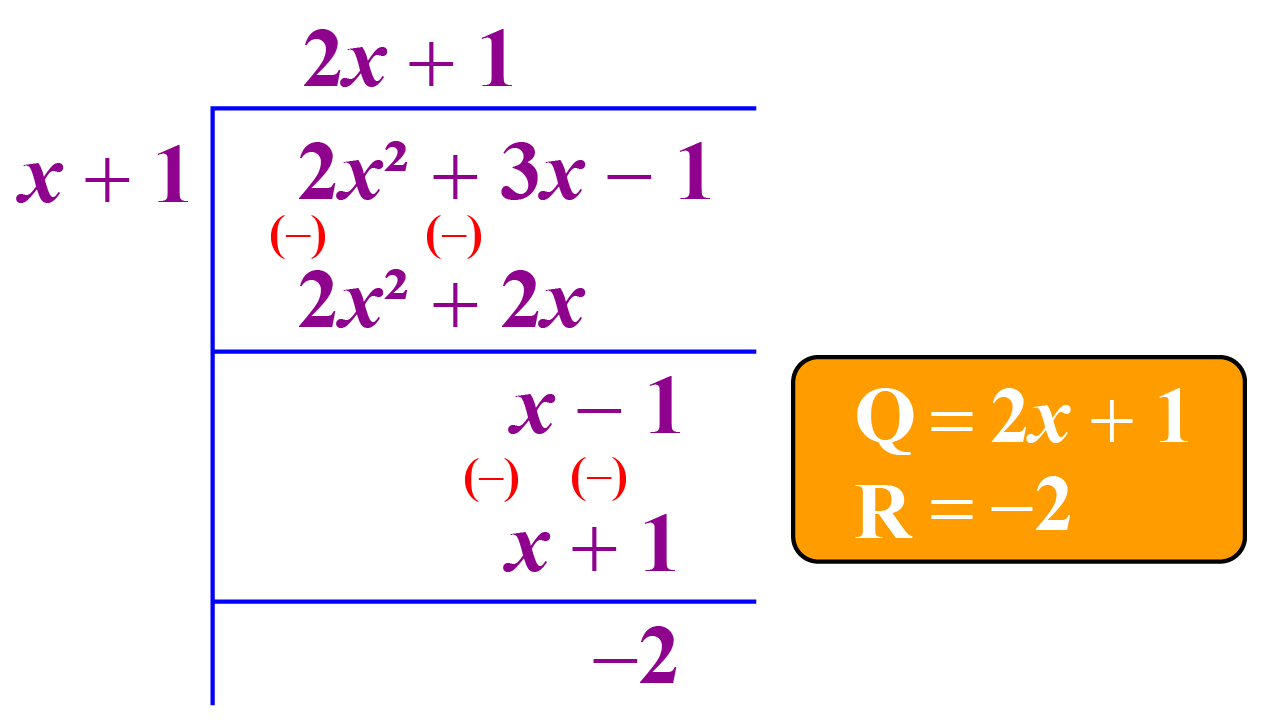When a polynomial $$P(x)$$ is to be divided by a linear factor, we write the coefficients alone, bring down the first coefficient, multiply, and add.

Repeat the multiplication and addition until we reach the end term of the polynomial.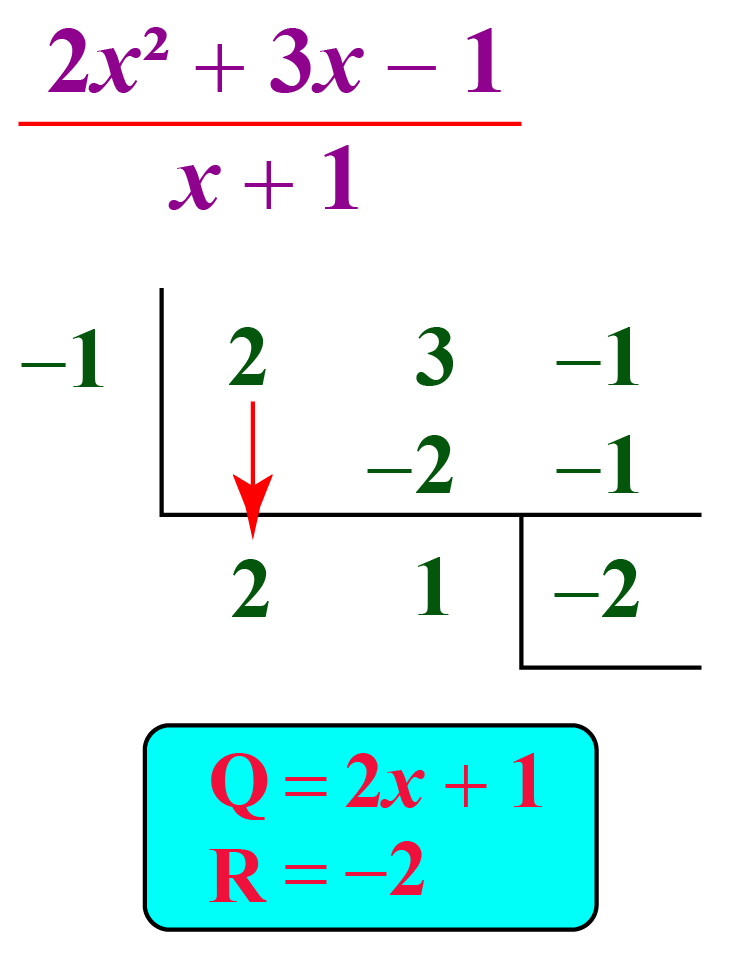Using synthetic division, we can perform complex division and obtain the solutions easily.

## How to Perform Synthetic Division of Polynomials?

Synthetic division of polynomials uses numbers for calculation and avoids the usage of variables.

In the place of division, we multiply, and in the place of subtraction, we add.

### Synthetic Division Quotient

• Write the coefficients of the dividend and use the zero of the linear factor in the divisor's place.
• Bring the first coefficient down and multiply it with the divisor.
• Write the product below the 2nd coefficient and add the column.
• Repeat until the last coefficient. The last number is taken as the remainder.
• Take the coefficients and write the quotient.
• Note that the resultant polynomial is of one order less than the dividend polynomial.

### Synthetic Division of Polynomials Examples

1) Consider this division: $$\dfrac{x^3 - 2x^2 -8x -35}{x-5}$$

The polynomial is of order 3

The divisor is a linear factor.

Let's use synthetic division to find the quotient.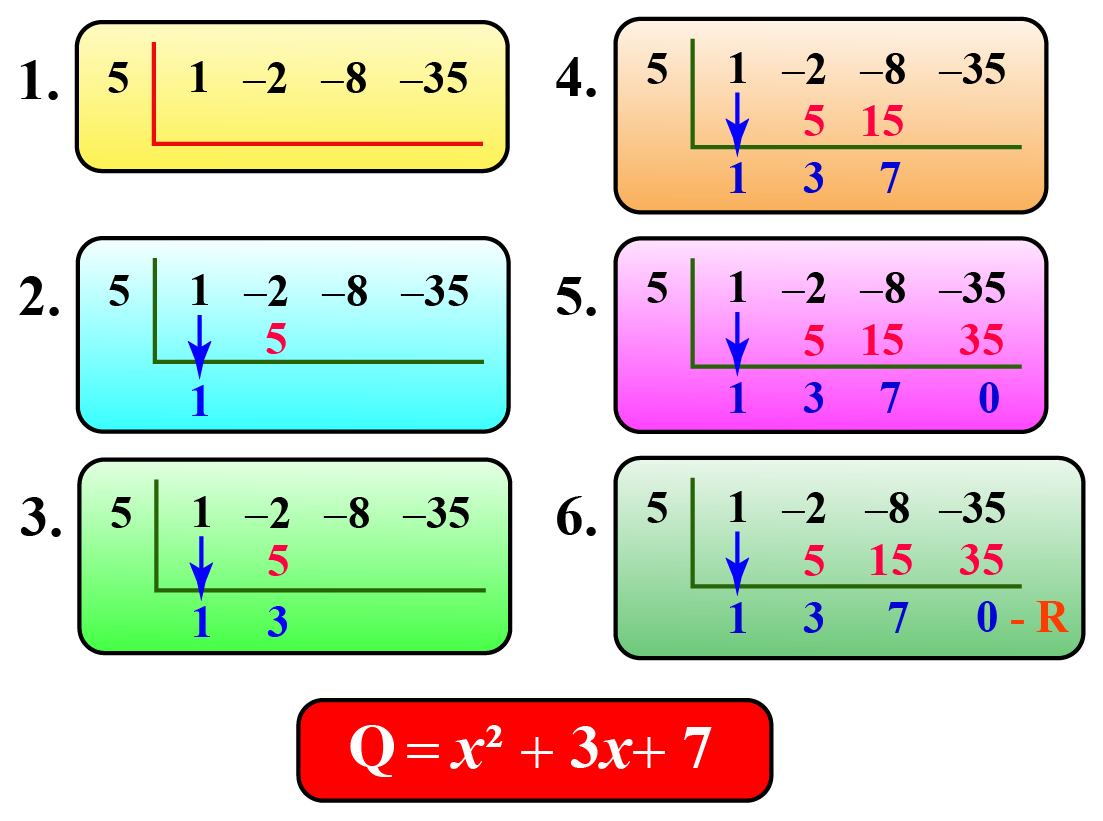Thus, the quotient is one order less than the given polynomial.

It is $$x^2 +3x +7$$ and the remainder is 0

$\dfrac{x^3 - 2x^2 -8x -35}{x-5} = x^2 +3x +7$

2) Consider this division: $\dfrac{6x^2+7x-20}{2x+5}$

We find the zero of the linear factor is $$\dfrac{-5}{2}$$.

We use synthetic division and get a row of numbers.

$$\therefore$$ After grouping the quotient, we will divide it by 2

###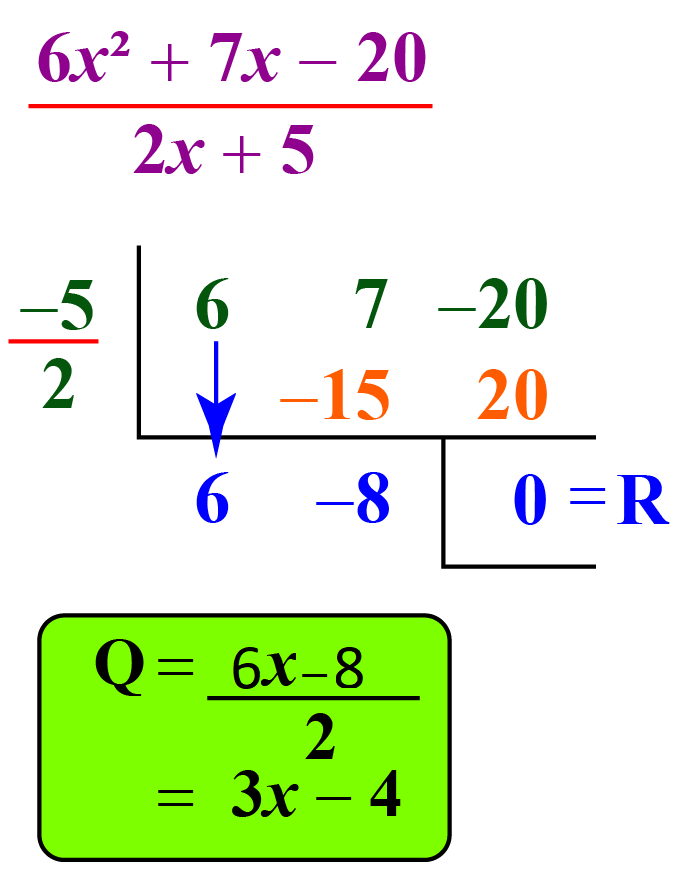Thus, the quotient is $$3x-4$$ and the remainder is 0

$\dfrac{6x^2+7x-20}{2x+5} = 3x-4$Tips and Tricks

• Perform synthetic division only when the divisor is a linear factor.
• Perform multiplication and addition in the place of division and subtraction that is used in the long division method.

## What Are the Steps for Synthetic Division of Polynomials?

The following are the steps while performing synthetic division and finding the quotient and the remainder.

• Check whether the polynomial is in the standard form.
• Write the coefficients in the dividend's place.
• Write the zero of the linear factor in the divisor's place.
• Bring the first coefficient down.
• Multiply it with the divisor and write it below the next coefficient.
• Add them and write the value below.
• Repeat the previous 2 steps until you reach the last term.
• Separate the last term thus obtained which is the remainder.
• Now group the coefficients with the variables to get the quotient.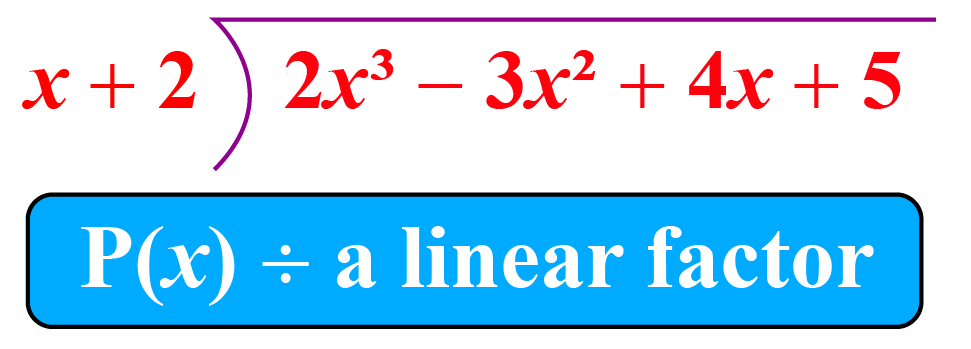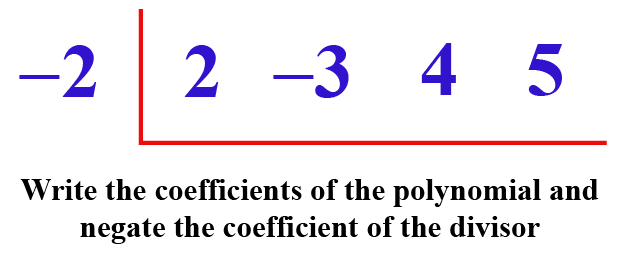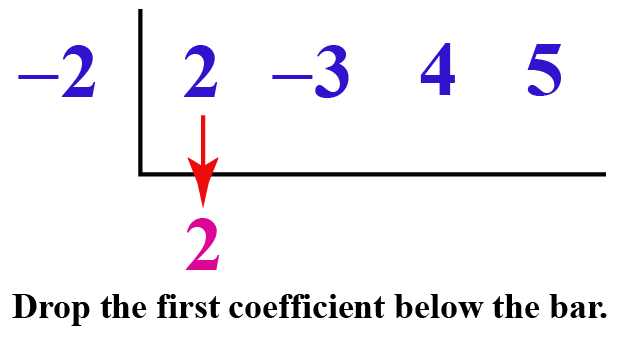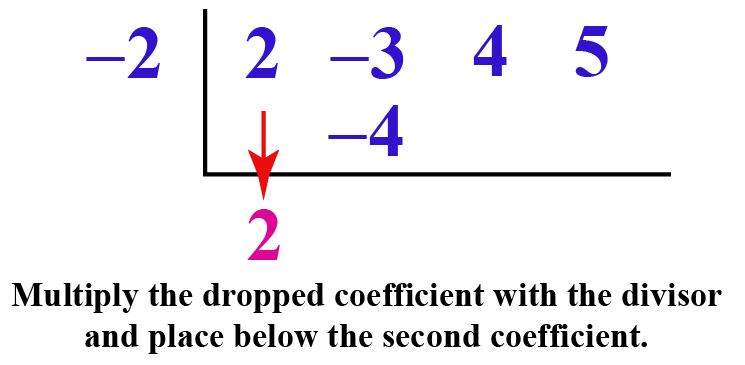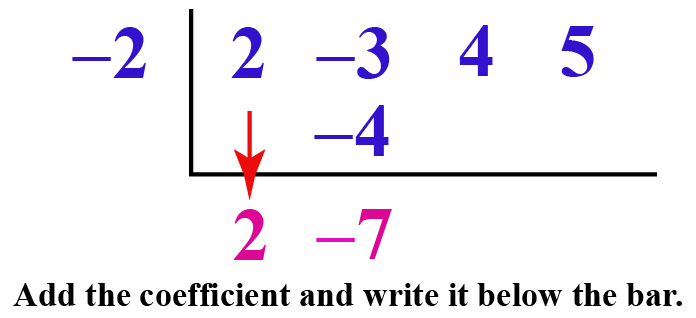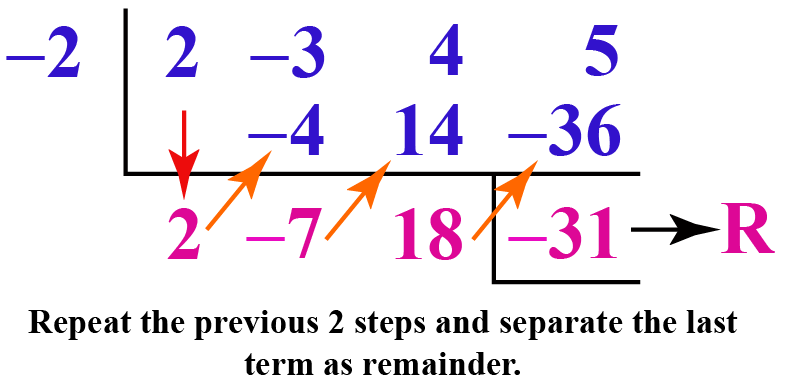### Synthetic Division Calculator

In the following simulation, let's recall the technique to divide using synthetic division.

Choose the order of the polynomial and enter the coefficients of the dividend polynomial and give the value of the linear factor.

You can also verify your resultant polynomial using the long division method.

## Solved Examples

 Example 1

The distance covered by Steve in his car is given by the expression $$9a^2-39a - 30$$.

The time taken by him to cover this distance is given by the expression $$(a - 5)$$.

Find the speed of the car.

Solution

Speed is given as the ratio of the distance to the time.

Speed = $\dfrac{9a^2 - 39a - 30}{a- 5}$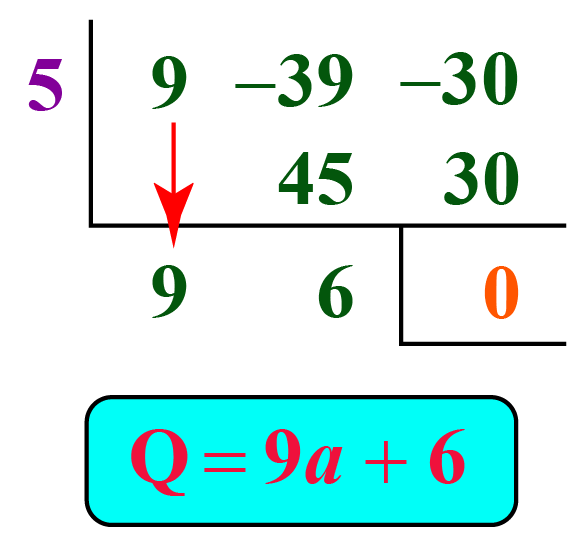Speed = $$9a + 6$$

 $$\therefore$$ Speed is given by the expression $$9a+6$$
 Example 2

The volume of Sara's storage box is $$8x ^3+12x ^2 - 2x - 3$$.

She knows that the area of the box is $$4x^2-1$$.

What could be the height of the box?

Solution

$\text{Area (A)} = \text{length(l)} \times \text{breadth(b)}$

Given $$A = 4x^2-1$$. This is of the form $$a^2 - b^2= (a+b) (a-b)$$

Thus, length and breadth are $$(2x+1)(2x-1)$$

\begin{align}\text{V} &= \text{l} \times \text{b}\times \text{h}\\\\&=\text{A} \times \text{h}\\\\ \text{h}&= \dfrac{V}{A}\\\\&=\dfrac{8x ^3+12x ^2 - 2x - 3}{((2x+1)(2x-1))}\end{align}

Let's solve this by the synthetic division twice.

/* 8 should be brought down; not 6 in the first step. */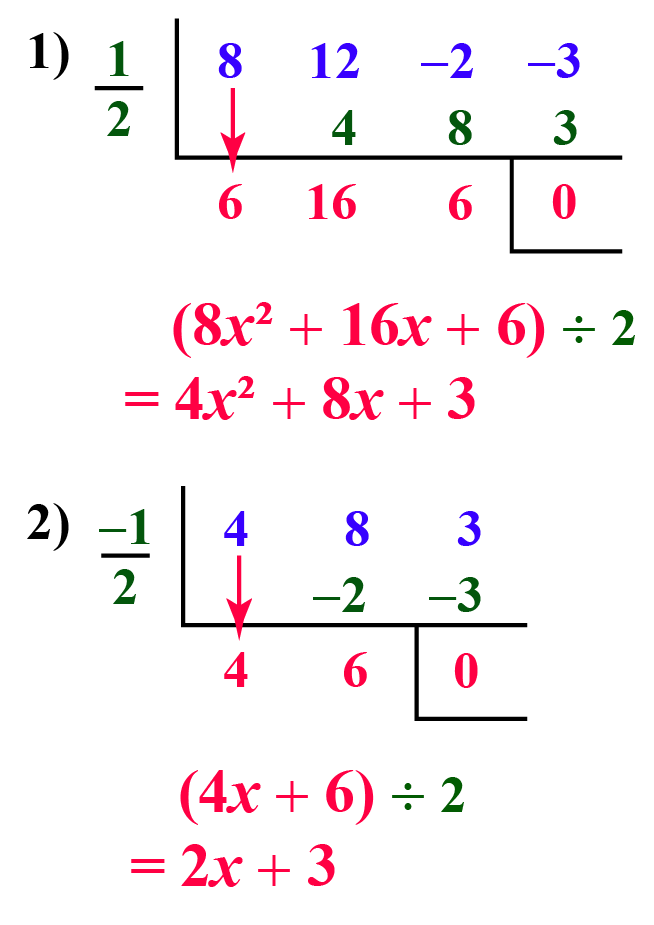$$\therefore$$Height of the box = $$2x+3$$

## Interactive Questions

Here are a few activities for you to practice. Select/Type your answer and click the "Check Answer" button to see the result.

## Let's Summarize

The mini-lesson targeted the fascinating concept of synthetic division of polynomials. The math journey around synthetic division started with what a student already knew and went on to creatively crafting a fresh concept in the young minds. Done in a way that not only it is relatable and easy to grasp, but also will stay with them forever.

At Cuemath, our team of math experts is dedicated to making learning fun for our favorite readers, the students!

Through an interactive and engaging learning-teaching-learning approach, the teachers explore all angles of a topic.

Be it worksheets, online classes, doubt sessions, or any other form of relation, it’s the logical thinking and smart learning approach that we, at Cuemath, believe in.

### 1. What do you mean by synthetic division of polynomials?

When a polynomial has to be divided by a linear factor, synthetic division is the shortest method.

### 2. How do you divide polynomials by synthetic division?

Take the coefficients alone, bring the first down, multiply with the zero of the linear factor, and add with the next coefficient and repeat until the end.

### 3. What is the importance of the synthetic division of polynomials?

Synthetic division can be generalized and expanded to the division of any polynomial with any polynomial.

### 4. What are the advantages of the synthetic division of polynomials?

This method uses fewer calculations and is quicker than long division.

### 5. What are the disadvantages of synthetic division of polynomials?

The synthetic division can be used only when the divisor is a linear polynomial.

### 6. What are the main uses of synthetic division of polynomials?

Synthetic division of polynomials help in finding the zeros of the polynomial.

### 7. What is the quotient in synthetic division?

In synthetic division, the polynomial obtained is one power lesser than the power of the dividend polynomial.

More Important Topics
Numbers
Algebra
Geometry
Measurement
Money
Data
Trigonometry
Calculus